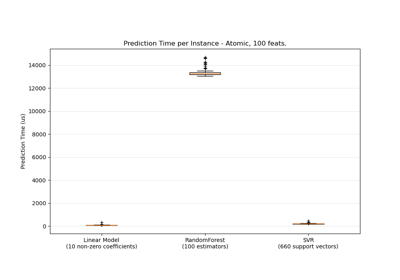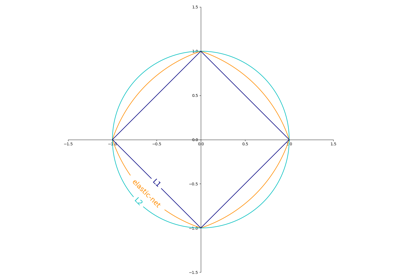# sklearn.linear_model.SGDRegressor¶

class sklearn.linear_model.SGDRegressor(loss='squared_error', *, penalty='l2', alpha=0.0001, l1_ratio=0.15, fit_intercept=True, max_iter=1000, tol=0.001, shuffle=True, verbose=0, epsilon=0.1, random_state=None, learning_rate='invscaling', eta0=0.01, power_t=0.25, early_stopping=False, validation_fraction=0.1, n_iter_no_change=5, warm_start=False, average=False)[source]

Linear model fitted by minimizing a regularized empirical loss with SGD.

SGD stands for Stochastic Gradient Descent: the gradient of the loss is estimated each sample at a time and the model is updated along the way with a decreasing strength schedule (aka learning rate).

The regularizer is a penalty added to the loss function that shrinks model parameters towards the zero vector using either the squared euclidean norm L2 or the absolute norm L1 or a combination of both (Elastic Net). If the parameter update crosses the 0.0 value because of the regularizer, the update is truncated to 0.0 to allow for learning sparse models and achieve online feature selection.

This implementation works with data represented as dense numpy arrays of floating point values for the features.

Read more in the User Guide.

Parameters:
lossstr, default=’squared_error’

The loss function to be used. The possible values are ‘squared_error’, ‘huber’, ‘epsilon_insensitive’, or ‘squared_epsilon_insensitive’

The ‘squared_error’ refers to the ordinary least squares fit. ‘huber’ modifies ‘squared_error’ to focus less on getting outliers correct by switching from squared to linear loss past a distance of epsilon. ‘epsilon_insensitive’ ignores errors less than epsilon and is linear past that; this is the loss function used in SVR. ‘squared_epsilon_insensitive’ is the same but becomes squared loss past a tolerance of epsilon.

More details about the losses formulas can be found in the User Guide.

penalty{‘l2’, ‘l1’, ‘elasticnet’, None}, default=’l2’

The penalty (aka regularization term) to be used. Defaults to ‘l2’ which is the standard regularizer for linear SVM models. ‘l1’ and ‘elasticnet’ might bring sparsity to the model (feature selection) not achievable with ‘l2’. No penalty is added when set to None.

alphafloat, default=0.0001

Constant that multiplies the regularization term. The higher the value, the stronger the regularization. Also used to compute the learning rate when set to learning_rate is set to ‘optimal’.

l1_ratiofloat, default=0.15

The Elastic Net mixing parameter, with 0 <= l1_ratio <= 1. l1_ratio=0 corresponds to L2 penalty, l1_ratio=1 to L1. Only used if penalty is ‘elasticnet’.

fit_interceptbool, default=True

Whether the intercept should be estimated or not. If False, the data is assumed to be already centered.

max_iterint, default=1000

The maximum number of passes over the training data (aka epochs). It only impacts the behavior in the fit method, and not the partial_fit method.

New in version 0.19.

tolfloat or None, default=1e-3

The stopping criterion. If it is not None, training will stop when (loss > best_loss - tol) for n_iter_no_change consecutive epochs. Convergence is checked against the training loss or the validation loss depending on the early_stopping parameter.

New in version 0.19.

shufflebool, default=True

Whether or not the training data should be shuffled after each epoch.

verboseint, default=0

The verbosity level.

epsilonfloat, default=0.1

Epsilon in the epsilon-insensitive loss functions; only if loss is ‘huber’, ‘epsilon_insensitive’, or ‘squared_epsilon_insensitive’. For ‘huber’, determines the threshold at which it becomes less important to get the prediction exactly right. For epsilon-insensitive, any differences between the current prediction and the correct label are ignored if they are less than this threshold.

random_stateint, RandomState instance, default=None

Used for shuffling the data, when shuffle is set to True. Pass an int for reproducible output across multiple function calls. See Glossary.

learning_ratestr, default=’invscaling’

The learning rate schedule:

• ‘constant’: eta = eta0

• ‘optimal’: eta = 1.0 / (alpha * (t + t0)) where t0 is chosen by a heuristic proposed by Leon Bottou.

• ‘invscaling’: eta = eta0 / pow(t, power_t)

• ‘adaptive’: eta = eta0, as long as the training keeps decreasing. Each time n_iter_no_change consecutive epochs fail to decrease the training loss by tol or fail to increase validation score by tol if early_stopping is True, the current learning rate is divided by 5.

eta0float, default=0.01

The initial learning rate for the ‘constant’, ‘invscaling’ or ‘adaptive’ schedules. The default value is 0.01.

power_tfloat, default=0.25

The exponent for inverse scaling learning rate.

early_stoppingbool, default=False

Whether to use early stopping to terminate training when validation score is not improving. If set to True, it will automatically set aside a fraction of training data as validation and terminate training when validation score returned by the score method is not improving by at least tol for n_iter_no_change consecutive epochs.

New in version 0.20: Added ‘early_stopping’ option

validation_fractionfloat, default=0.1

The proportion of training data to set aside as validation set for early stopping. Must be between 0 and 1. Only used if early_stopping is True.

New in version 0.20: Added ‘validation_fraction’ option

n_iter_no_changeint, default=5

Number of iterations with no improvement to wait before stopping fitting. Convergence is checked against the training loss or the validation loss depending on the early_stopping parameter.

New in version 0.20: Added ‘n_iter_no_change’ option

warm_startbool, default=False

When set to True, reuse the solution of the previous call to fit as initialization, otherwise, just erase the previous solution. See the Glossary.

Repeatedly calling fit or partial_fit when warm_start is True can result in a different solution than when calling fit a single time because of the way the data is shuffled. If a dynamic learning rate is used, the learning rate is adapted depending on the number of samples already seen. Calling fit resets this counter, while partial_fit will result in increasing the existing counter.

averagebool or int, default=False

When set to True, computes the averaged SGD weights across all updates and stores the result in the coef_ attribute. If set to an int greater than 1, averaging will begin once the total number of samples seen reaches average. So average=10 will begin averaging after seeing 10 samples.

Attributes:
coef_ndarray of shape (n_features,)

Weights assigned to the features.

intercept_ndarray of shape (1,)

The intercept term.

n_iter_int

The actual number of iterations before reaching the stopping criterion.

t_int

Number of weight updates performed during training. Same as (n_iter_ * n_samples + 1).

n_features_in_int

Number of features seen during fit.

New in version 0.24.

feature_names_in_ndarray of shape (n_features_in_,)

Names of features seen during fit. Defined only when X has feature names that are all strings.

New in version 1.0.

HuberRegressor

Linear regression model that is robust to outliers.

Lars

Least Angle Regression model.

Lasso

Linear Model trained with L1 prior as regularizer.

RANSACRegressor

RANSAC (RANdom SAmple Consensus) algorithm.

Ridge

Linear least squares with l2 regularization.

sklearn.svm.SVR

Epsilon-Support Vector Regression.

TheilSenRegressor

Theil-Sen Estimator robust multivariate regression model.

Examples

>>> import numpy as np
>>> from sklearn.linear_model import SGDRegressor
>>> from sklearn.pipeline import make_pipeline
>>> from sklearn.preprocessing import StandardScaler
>>> n_samples, n_features = 10, 5
>>> rng = np.random.RandomState(0)
>>> y = rng.randn(n_samples)
>>> X = rng.randn(n_samples, n_features)
>>> # Always scale the input. The most convenient way is to use a pipeline.
>>> reg = make_pipeline(StandardScaler(),
...                     SGDRegressor(max_iter=1000, tol=1e-3))
>>> reg.fit(X, y)
Pipeline(steps=[('standardscaler', StandardScaler()),
('sgdregressor', SGDRegressor())])


Methods

 Convert coefficient matrix to dense array format. fit(X, y[, coef_init, intercept_init, ...]) Fit linear model with Stochastic Gradient Descent. get_params([deep]) Get parameters for this estimator. partial_fit(X, y[, sample_weight]) Perform one epoch of stochastic gradient descent on given samples. Predict using the linear model. score(X, y[, sample_weight]) Return the coefficient of determination of the prediction. set_params(**params) Set the parameters of this estimator. Convert coefficient matrix to sparse format.
densify()[source]

Convert coefficient matrix to dense array format.

Converts the coef_ member (back) to a numpy.ndarray. This is the default format of coef_ and is required for fitting, so calling this method is only required on models that have previously been sparsified; otherwise, it is a no-op.

Returns:
self

Fitted estimator.

fit(X, y, coef_init=None, intercept_init=None, sample_weight=None)[source]

Fit linear model with Stochastic Gradient Descent.

Parameters:
X{array-like, sparse matrix}, shape (n_samples, n_features)

Training data.

yndarray of shape (n_samples,)

Target values.

coef_initndarray of shape (n_features,), default=None

The initial coefficients to warm-start the optimization.

intercept_initndarray of shape (1,), default=None

The initial intercept to warm-start the optimization.

sample_weightarray-like, shape (n_samples,), default=None

Weights applied to individual samples (1. for unweighted).

Returns:
selfobject

Fitted SGDRegressor estimator.

get_params(deep=True)[source]

Get parameters for this estimator.

Parameters:
deepbool, default=True

If True, will return the parameters for this estimator and contained subobjects that are estimators.

Returns:
paramsdict

Parameter names mapped to their values.

partial_fit(X, y, sample_weight=None)[source]

Perform one epoch of stochastic gradient descent on given samples.

Internally, this method uses max_iter = 1. Therefore, it is not guaranteed that a minimum of the cost function is reached after calling it once. Matters such as objective convergence and early stopping should be handled by the user.

Parameters:
X{array-like, sparse matrix}, shape (n_samples, n_features)

Subset of training data.

ynumpy array of shape (n_samples,)

Subset of target values.

sample_weightarray-like, shape (n_samples,), default=None

Weights applied to individual samples. If not provided, uniform weights are assumed.

Returns:
selfobject

Returns an instance of self.

predict(X)[source]

Predict using the linear model.

Parameters:
X{array-like, sparse matrix}, shape (n_samples, n_features)

Input data.

Returns:
ndarray of shape (n_samples,)

Predicted target values per element in X.

score(X, y, sample_weight=None)[source]

Return the coefficient of determination of the prediction.

The coefficient of determination $$R^2$$ is defined as $$(1 - \frac{u}{v})$$, where $$u$$ is the residual sum of squares ((y_true - y_pred)** 2).sum() and $$v$$ is the total sum of squares ((y_true - y_true.mean()) ** 2).sum(). The best possible score is 1.0 and it can be negative (because the model can be arbitrarily worse). A constant model that always predicts the expected value of y, disregarding the input features, would get a $$R^2$$ score of 0.0.

Parameters:
Xarray-like of shape (n_samples, n_features)

Test samples. For some estimators this may be a precomputed kernel matrix or a list of generic objects instead with shape (n_samples, n_samples_fitted), where n_samples_fitted is the number of samples used in the fitting for the estimator.

yarray-like of shape (n_samples,) or (n_samples, n_outputs)

True values for X.

sample_weightarray-like of shape (n_samples,), default=None

Sample weights.

Returns:
scorefloat

$$R^2$$ of self.predict(X) w.r.t. y.

Notes

The $$R^2$$ score used when calling score on a regressor uses multioutput='uniform_average' from version 0.23 to keep consistent with default value of r2_score. This influences the score method of all the multioutput regressors (except for MultiOutputRegressor).

set_params(**params)[source]

Set the parameters of this estimator.

The method works on simple estimators as well as on nested objects (such as Pipeline). The latter have parameters of the form <component>__<parameter> so that it’s possible to update each component of a nested object.

Parameters:
**paramsdict

Estimator parameters.

Returns:
selfestimator instance

Estimator instance.

sparsify()[source]

Convert coefficient matrix to sparse format.

Converts the coef_ member to a scipy.sparse matrix, which for L1-regularized models can be much more memory- and storage-efficient than the usual numpy.ndarray representation.

The intercept_ member is not converted.

Returns:
self

Fitted estimator.

Notes

For non-sparse models, i.e. when there are not many zeros in coef_, this may actually increase memory usage, so use this method with care. A rule of thumb is that the number of zero elements, which can be computed with (coef_ == 0).sum(), must be more than 50% for this to provide significant benefits.

After calling this method, further fitting with the partial_fit method (if any) will not work until you call densify.

## Examples using sklearn.linear_model.SGDRegressor¶Prediction Latency

Prediction LatencySGD: Penalties

SGD: Penalties